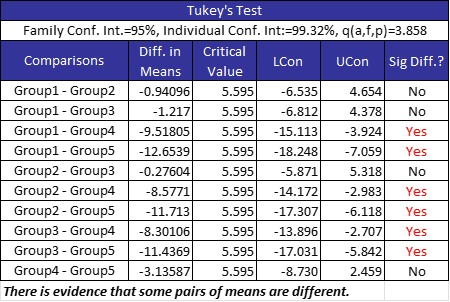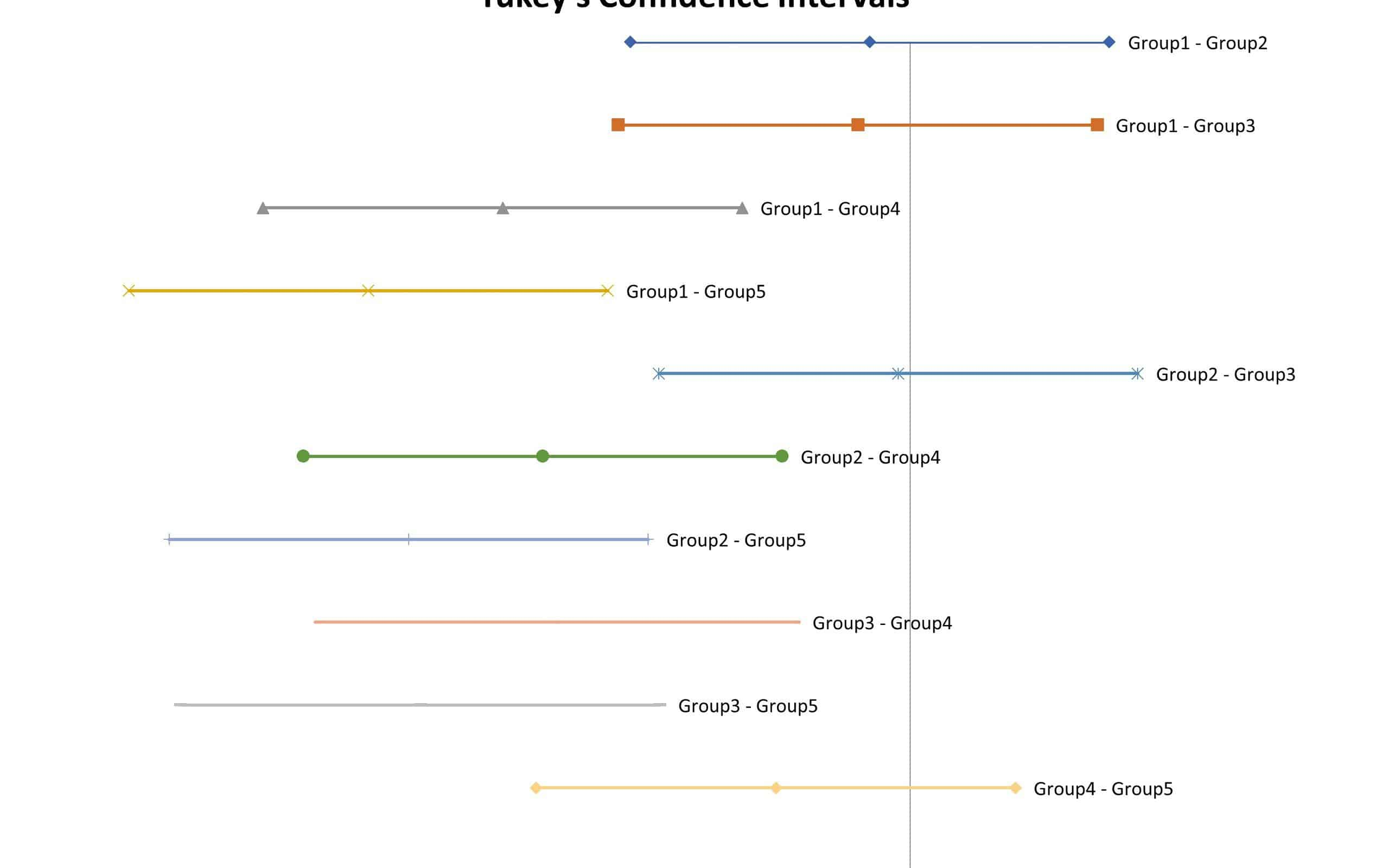ANOVA will tell you whether there is a statistically significant difference in the population means of three or more groups of data. But which means are different? Tukey’s will tell you that.

Analysis of Variance (ANOVA) is used to determine whether the population means of several sample data sets are statistically different or not. If they are different, which ones are different from each other? Tukey’s multiple comparison test, developed by statistician John Tukey, is also called Tukey’s honestly significant difference test or Tukey’s HSD.

Tukey’s method is often used as a post hoc test for an ANOVA, to create confidence intervals for all pairwise differences between each group’s means, while controlling the overall error rate to a level that you choose.

## Overview: What is Tukey’s?

After you have run an ANOVA and found significant results, then you can run Tukey’s HSD to find out which specific group means, when compared with each other, are different. The test compares all possible pairs of group means.

There are several assumptions you need to meet before doing your Tukey test:

One of the downsides of doing multiple comparison tests is that the overall Type 1 error rate will be inflated. This family-wise error rate must be controlled to have meaningful results. In Tukey’s test results, the formula indicates how large an observed difference must be for the multiple comparison procedure to call it significant. Any absolute difference between means has to exceed the value of HSD to be statistically significant. You get to choose your error rate.

The Tukey test output is usually a series of confidence intervals of the difference in means between the combinations of groups. If that confidence interval contains the value of zero, you will interpret that to mean the two group means you compared are not statistically different.

## An industry example of Tukey’s

The manager of manufacturing wanted to determine whether the run speeds of his five machines were equal. He was advised to use an ANOVA to determine if there was any difference. The p-value from the ANOVA indicated the null hypothesis of no difference had to be rejected. He then used Tukey’s to determine which machines were different from each other.

Below you can see the results in table format and graphical format. In the graphical format, if the vertical line of zero falls within a paired comparison of two machines, then there is no statistically significant difference between the two machine speeds. The other confidence intervals will show the range of possible machine speed differences.### What is Tukey’s used for?

Tukey’s is one of several post hoc tests when your ANOVA test indicates there is a statistically significant difference in population means of several groups of data you are analyzing. Tukey’s identifies which means are different from the others.

### What do the confidence intervals in Tukey’s mean?

Tukey’s will calculate the confidence interval by doing a pairwise comparison of all the group means. The confidence interval (commonly 95%) shows the range of possible values for the difference between two group means. If the confidence interval contains zero, you can comfortably conclude the difference in means between the two groups is zero.

### Can Tukey’s be used for a non-parametric test?

No. Tukey’s is used for continuous data and is often a post hoc test for the parametric ANOVA test. There are no common equivalents to Tukey’s for non-parametric tests.